Function Repository Resource:

# EdgeMultiplicity

Count the multiplicity of edges in a graph

Contributed by: Nikolay Murzin
 ResourceFunction["EdgeMultiplicity"][graph] returns edge multiplicities for all the edges in a graph. ResourceFunction["EdgeMultiplicity"][graph, v] returns edge multiplicities for edges starting with vertex v. ResourceFunction["EdgeMultiplicity"][graph,All,w] returns edge multiplicities for edges ending with vertex w. ResourceFunction["EdgeMultiplicity"][graph,v,w] returns edge multiplicities starting with vertex v and ending with vertex w.

## Details

ResourceFunction["EdgeMultiplicity"] returns an Association with edges as keys and edge multiplicities as values.
The edge multiplicity of a given end vertex in a multigraph is the number of multiple edges sharing that end vertex.
For an undirected graph, an edge is taken to be both an in-edge and an out-edge.
ResourceFunction["EdgeMultiplicity"] works with undirected graphs, directed graphs, multigraphs and mixed graphs.

## Examples

### Basic Examples (3)

Count edges in an undirected multigraph:

 In:=Out=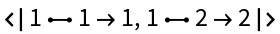Count edges in a directed multigraph:

 In:=Out=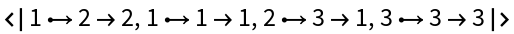Count edges in a mixed multigraph:

 In:=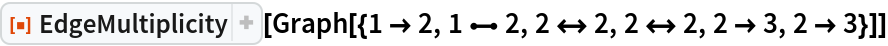Out=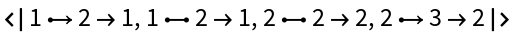### Scope (4)

Count edges starting with a given vertex:

 In:=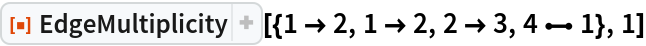Out=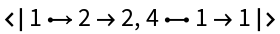Count edges ending with a given vertex:

 In:=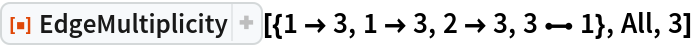Out=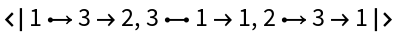Count edges starting and ending with a given vertex:

 In:=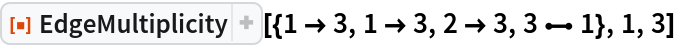Out=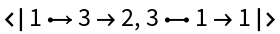Use patterns:

 In:=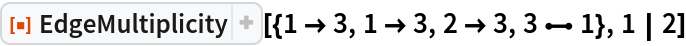Out=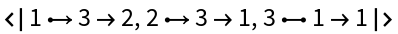## Version History

• 1.0.0 – 15 February 2022